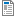National Curriculum

# Year 2/3 Maths Plans

Hamilton provide Mixed Year 2/3 weekly maths plans (below). We hope, in time, to develop maths blocks for this mixed year combination. Find out more about our plans to phase out these mixed age plans in favour of Year 2/3 maths blocks which we believe offer all the advantages of the weekly plans and more.

Supporting documents for set
• Week
• Title
1
+ Details
Number and place value

Year 2: Mark 2-digit numbers on a beaded line, then landmarked line. Order/compare numbers to 100. Say a number between neighbouring pairs of multiples of ten. Count on in tens and back again. Make sensible estimates. Partition 2-digit numbers; Use place value to add and subtract. Year 3: Place two and 3-digit numbers on a line. Understand place value in, order and compare 3-digit numbers. Use place value to add and subtract.Autumn Week 1 Plan
•Autumn Week 1 Image Resource
•ITP Number Grid
2
+ Details

Year 2:  Partition numbers to 10, then 20 into pairs, record related addition sentences and corresponding subtraction facts.;Recognise the use of a symbol such as ■ to represent an unknown; Add/subtract a single digit to/from a 2-digit number not crossing 10s, using number facts and pattern. Add three small numbers. Year 3:  Objectives: Know number bonds to 20 and use them in addition and subtraction; Use = to represent equality; Add and subtract 1-digit numbers to/from 2-digit numbers. Includes bar model examples.Autumn Week 2 Plan
3
+ Details

Year 2: Recognise all coins. Find totals up to 20p and change from 20p. Find all possibilities by making an ordered list. Use pairs to ten to find the complement to the next multiple of ten. Year 3: Understand and use place value with money using £ and p notation. Subtract pairs of 2-digit numbers by counting up. Find change from £1. Includes bar model examples.

4
+ Details
Time, position and direction

Year 2: Read the time to the quarter of an hour on digital and analogue clocks. Begin to identify time intervals. Identify left and right, recognise right angles, whole, half and quarter turns, both clockwise and anticlockwise. Year 3: Tell the time to five minutes using analogue, digital and Roman numeral clocks. Understand am and pm times. Understand angles as degrees of turn, right angles as quarter turns, clockwise and anticlockwise. Recognise that two right angles make a half-turn, three make three quarters of a turn and four a complete turn.

5
+ Details

Year 2: Count on in tens from any single-digit number then any number, and back again, relating this to finding 10 more/less. Add and subtract 10, 20, 11 and 21. Year 3: Add pairs of 2-digit numbers by partitioning. Use place value in money to add and subtract; Add/subtract 1, 10 and 100 to/from any 3-digit number.

6
+ Details
Multiplication, division and fractions

Year 2: Count in 10s from any number. Recognise multiples of 10. Begin to use multiplication. Count in 2s. Recognise odd and even numbers. Find halves and quarters of shapes. Year 3: Know × and ÷ facts for 2, 5 and 10 times tables. Understand multiplication is commutative. Recognise multiples of 2, 5 and 10. Understand concept of a fraction of a shape and quantity. Find ½, 1/3 and ¼ of quantities (whole number answers). Halve odd numbers. Includes bar model examples.

7
+ Details
Mental addition, subtraction, halving, doubling and fractions

Year 2: Find doubles to double 20 and corresponding halves. Add/subtract a single digit number to/from a 2-digit number by bridging multiples of ten using knowledge of pairs to ten and place value. Year 3: Double 2-digit numbers and halve even 2-digit numbers. Find fractions of a quantity: ½, ⅓, ¼, ¾, ⅔. Includes bar model examples.

8
+ Details

Year 2: Know pairs with a total of 20 and derive the subtraction facts. Recognise the use of a symbol such as ■ to represent an unknown. Use pairs to ten to find the complement to the next multiple of ten. Find change from 20p. Begin to find a difference between two numbers either side of a multiple of 10. Year 3: Know pairs of multiples of 5 and find other pairs of 2-digit numbers totalling 100. Subtract numbers on either side of 100 by counting up. Includes bar model examples.

9
+ Details
Length, capacity and place value in money

Year 2: Measure the length of objects using standard units. Estimate, measure and compare capacities, using suitable non-standard and standard units (litres). Year 3: Estimate, measure and convert lengths in cm and m, begin to measure in millimetres. Represent and interpret data in tables and bar charts; Understand and use place value in money, writing amounts in pounds and pence, adding and subtracting pounds, 10ps and 1ps, e.g. £4.63 – 60p and £3.49 + 30p.

10
+ Details